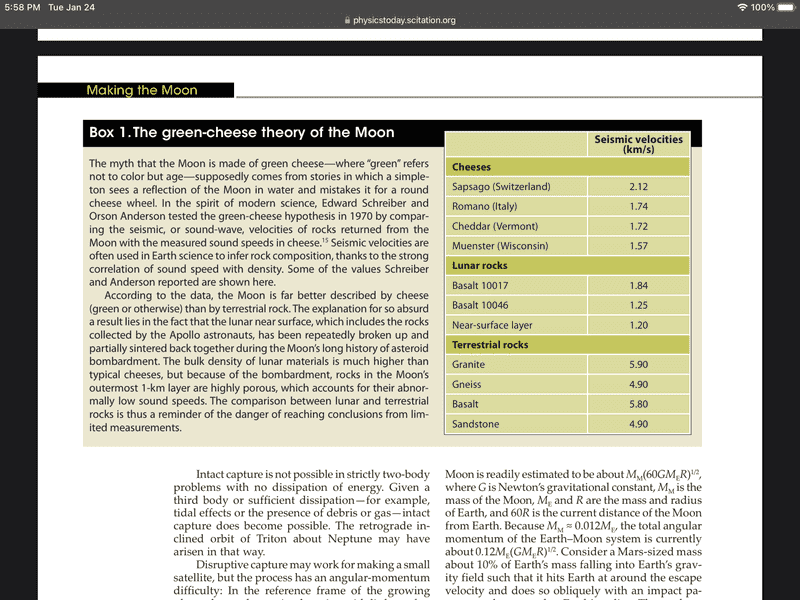# How do I find way of comparing the density of the Earth and the Moon?

DANIELWR1998
Homework Statement:
The radius of Earth is 4 times the radius of the moon. Estimate the acceleration due to gravity on the surface of the moon.

Do I use some orbital period information? I can't think of anything else that may be relevant.
Relevant Equations:
M = density x sphere volume
g = GM/r²
Surface acceleration is proportional to density and radius of planet (as 2 powers of R cancel with the volume)

Last edited:

Homework Helper
Gold Member
2022 Award
Hi @DANIELWR1998. Welcome to PF.

Note that question asks only for an 'Estimate'. This implies that an accurate answer is not required. If that's the case, you may be expected to assume that the densities of the earth and moon are equal. (If you do that, clearly state the assumption as part of your answer.)

Last edited by a moderator:
•DANIELWR1998
DANIELWR1998
Hi @DANIELWR1998. Welcome to PF.

Note that question asks only for an 'Estimate'. This implies that an accurate answer is not required. If that's the case, you may be expected to assume that the densities of the earth and moon are equal. (If you do that, clearly state the assumption as part of your answer.)
I did initially think that, but I know that the acceleration on the moon is less than 1/6 of Earth's. This assumption would give 1/4 as the answer which I'm not sure is accurate enough, I don't actually know whether it just wanted a very rough approximation.

Last edited by a moderator:
Homework Helper
Gold Member
2022 Award
I did initially think that, but I know that the acceleration on the moon is less than 1/6 of Earth's. This assumption would give 1/4 as the answer which I'm not sure is accurate enough, I don't actually know whether it just wanted a very rough approximation.
Consider giving 2 answers to cover yourself. The first uses the (stated) assumption of equal densities. For the second, look-up the densities of the moon and earth (give the references) and use them.

Your answer should include a proper derivation (using symbols/algebra) of any formula you end-up using. A phrase such as '2 powers of R cancel with the volume' doesn't really do the job!

Edit. Note that using 4 for the ratio of the radii isn't particularly accurate.

•DANIELWR1998
Homework Helper
Gold Member
2022 Award
I did initially think that, but I know that the acceleration on the moon is less than 1/6 of Earth's. This assumption would give 1/4 as the answer which I'm not sure is accurate enough, I don't actually know whether it just wanted a very rough approximation.
Why not solve the whole problem? All the information you need is on the Internet. Namely, the radius and density of the Earth and Moon. And, you can check the answer as the surface gravity can be found online as well.

This should give you a quantitative corroboration of Newton's law of gravity.

I can't see the point in the age of the Internet of doing anything else.

Homework Helper
Gold Member
2022 Award
This should give you a quantitative corroboration of Newton's law of gravity.
Not really. That law would surely have been assumed in obtaining the density estimate found on the internet.
I can't see the point in the age of the Internet of doing anything else.
By that reasoning, just look up the moon's surface gravity.

Though it doesn’t quite say it, I read the question as "The radius of Earth is 4 times the radius of the moon. On that basis, estimate the acceleration due to gravity on the surface of the moon in terms of g". So I would just assume the densities are similar.

••DANIELWR1998 and PeroK
DANIELWR1998
Not really. That law would surely have been assumed in obtaining the density estimate found on the internet.

By that reasoning, just look up the moon's surface gravity.

Though it doesn’t quite say it, I read the question as "The radius of Earth is 4 times the radius of the moon. On that basis, estimate the acceleration due to gravity on the surface of the moon in terms of g". So I would just assume the densities are similar.
I think this is the right reasoning based off of the answers so far. It came at the end of a University of Cambridge Natural Sciences Interview Preparation paper so I thought it would require a bit more than this as it's just one step (and what I assumed to begin with).

DANIELWR1998
Why not solve the whole problem? All the information you need is on the Internet. Namely, the radius and density of the Earth and Moon. And, you can check the answer as the surface gravity can be found online as well.

This should give you a quantitative corroboration of Newton's law of gravity.

I can't see the point in the age of the Internet of doing anything else.
It's more just an estimation problem but I thought the densities could be related using some other phenomenon such as an orbital period or if I knew the distance between the moon and the Earth. I wasn't quite sure what I was allowed to assume and what would be taken as common knowledge.

Gold Member
I thought it was made of green cheese so roughly 1-1.1 gm/ccPhysics Today 67, 11, 32 (2014)

Last edited:
••PeroK and kuruman
•